Polar representation

Chapter 5 Class 11 Complex Numbers
Concept wise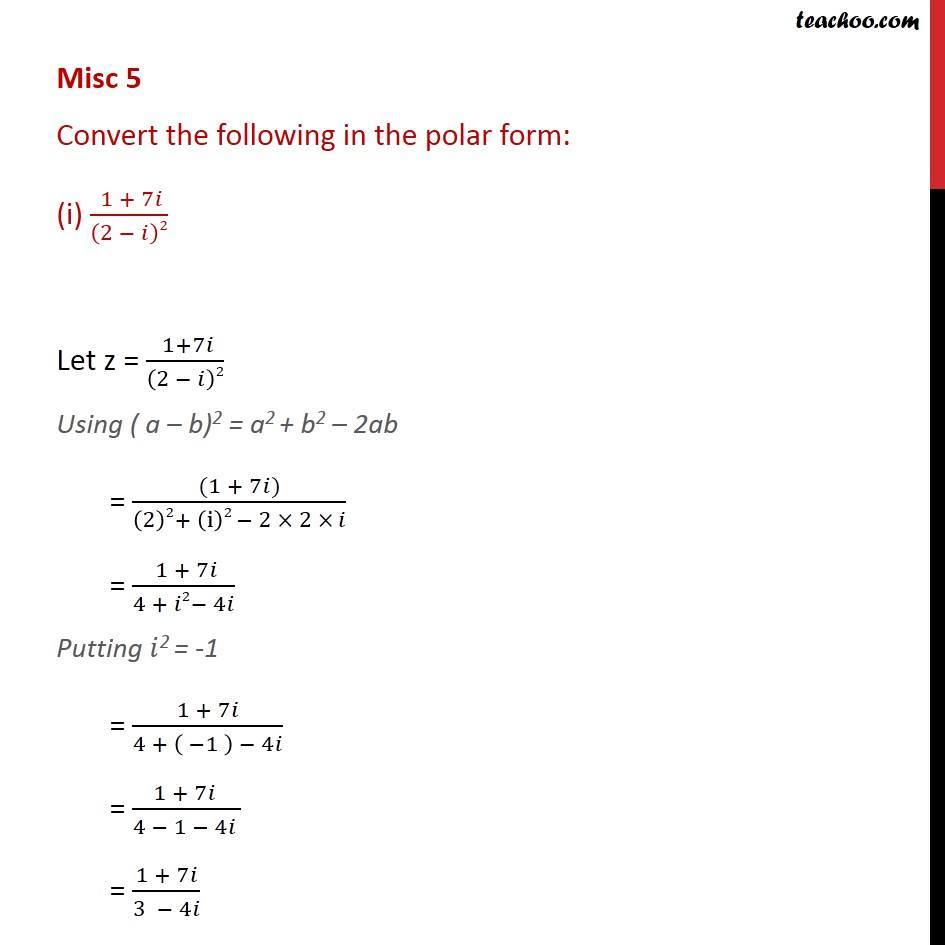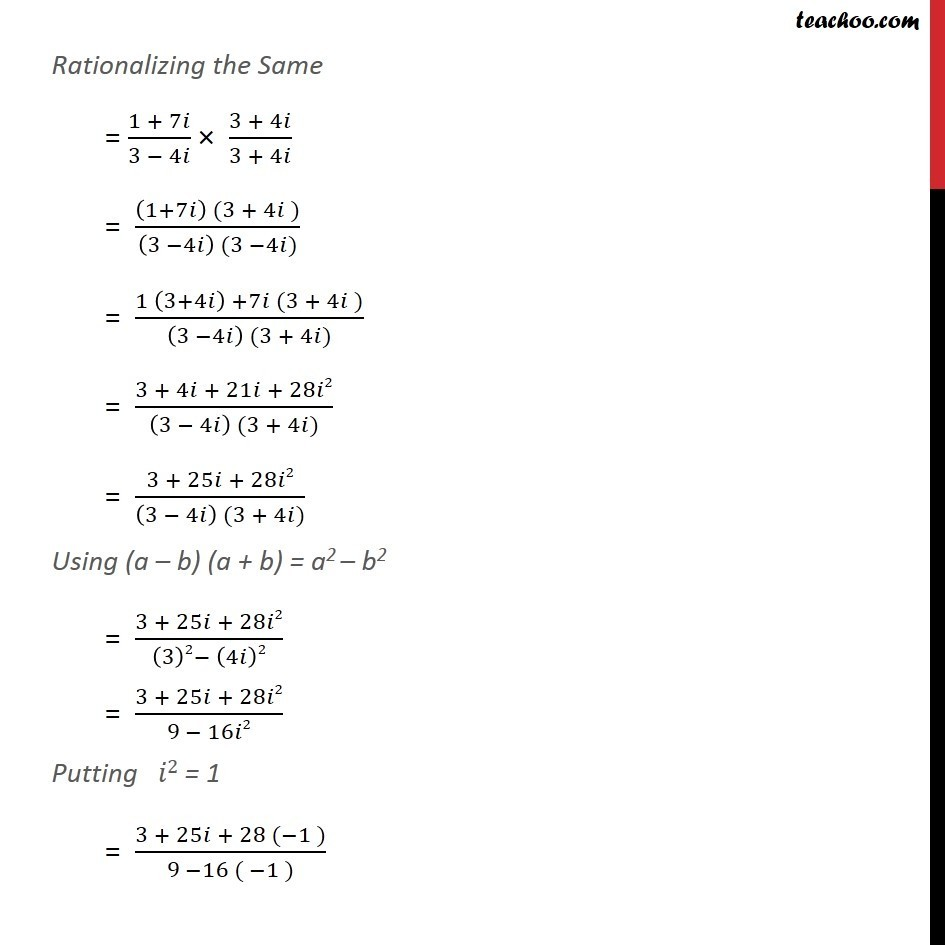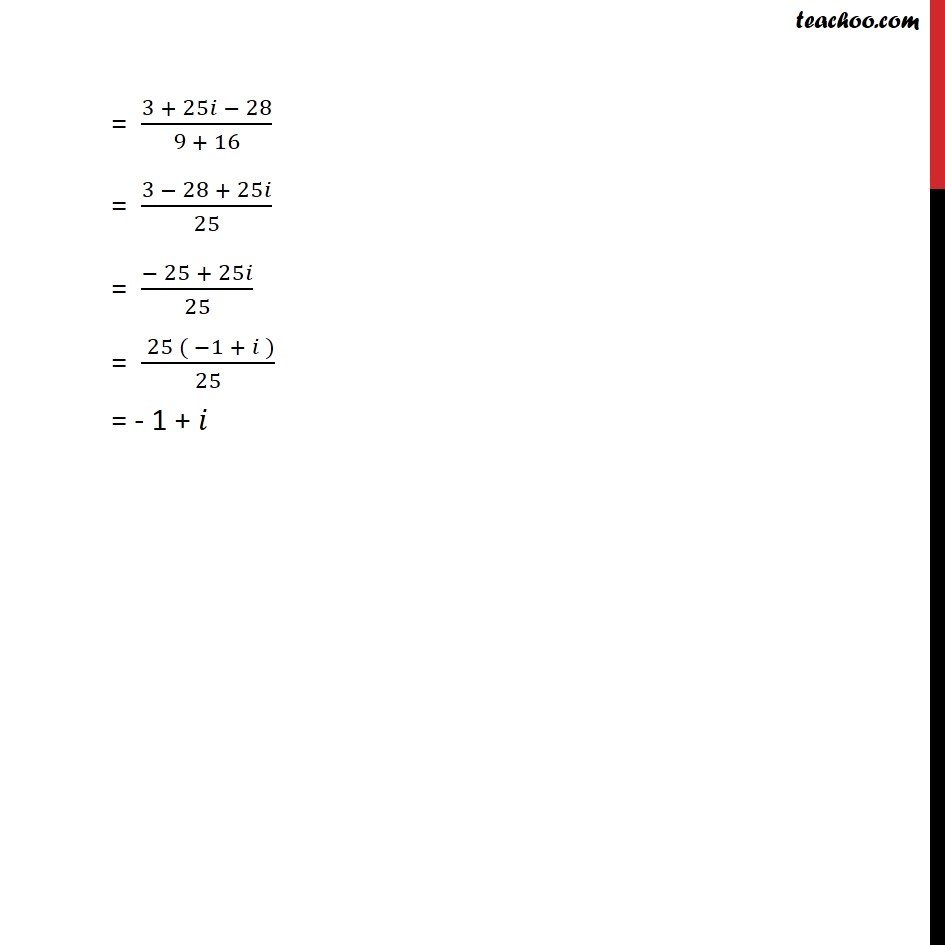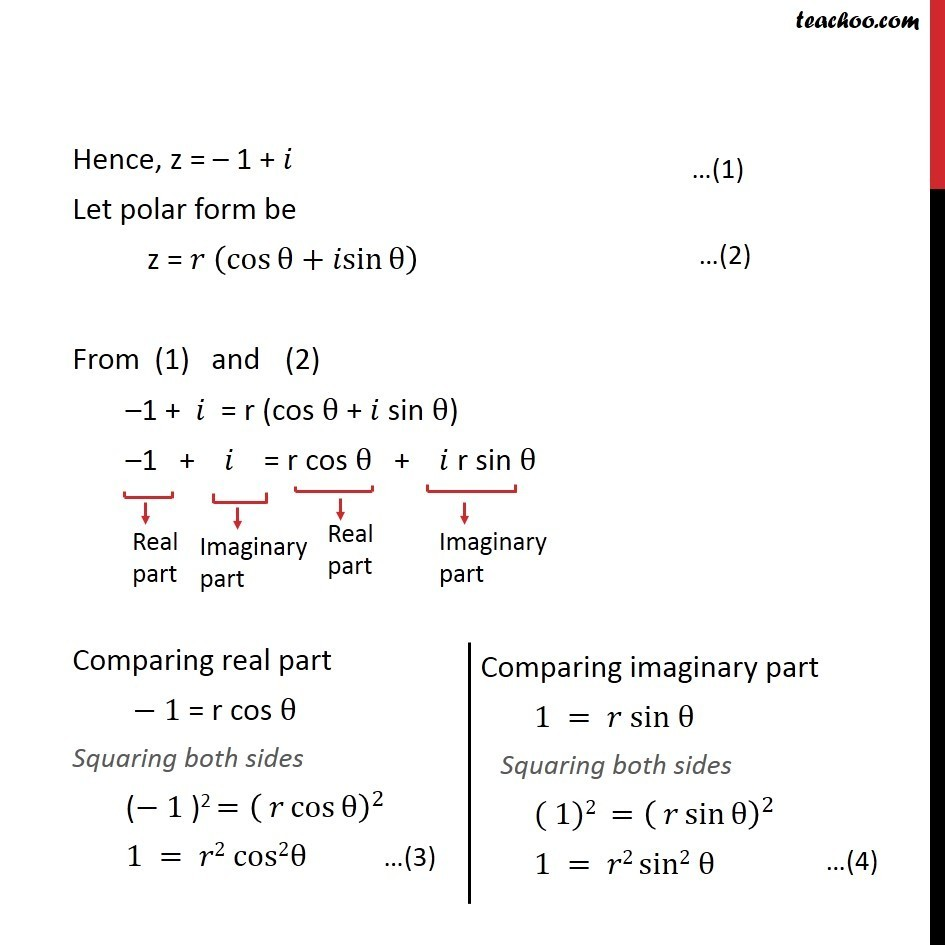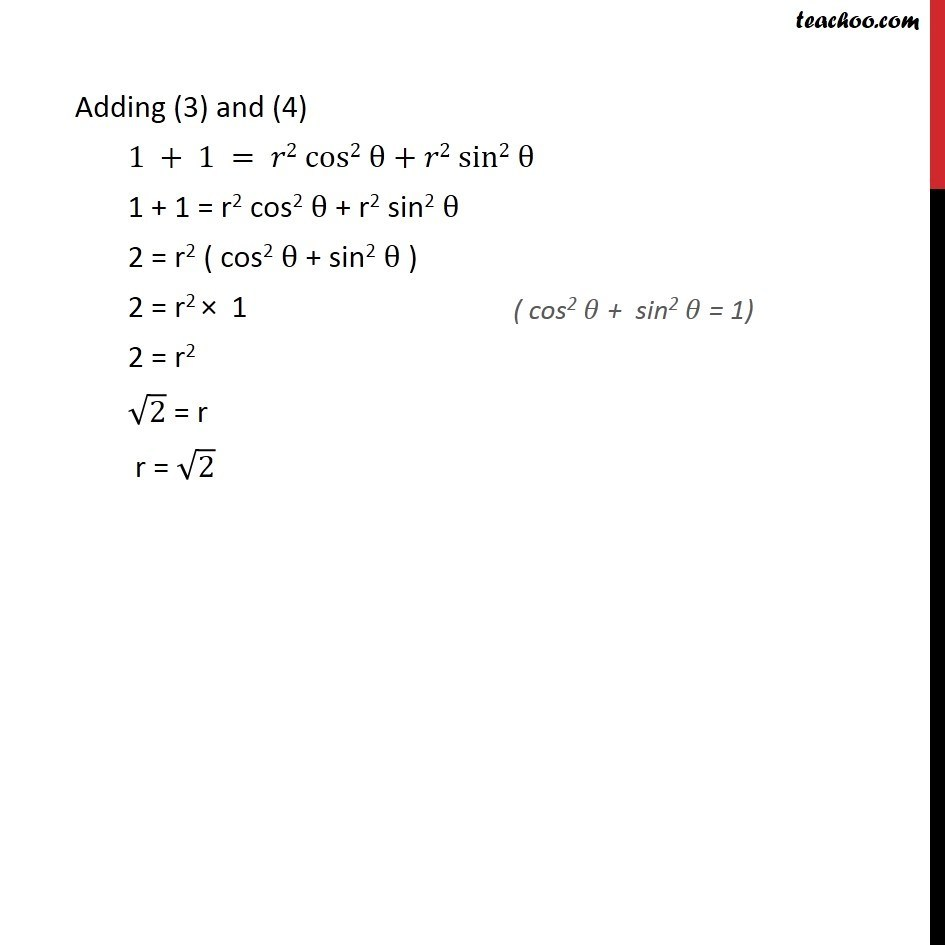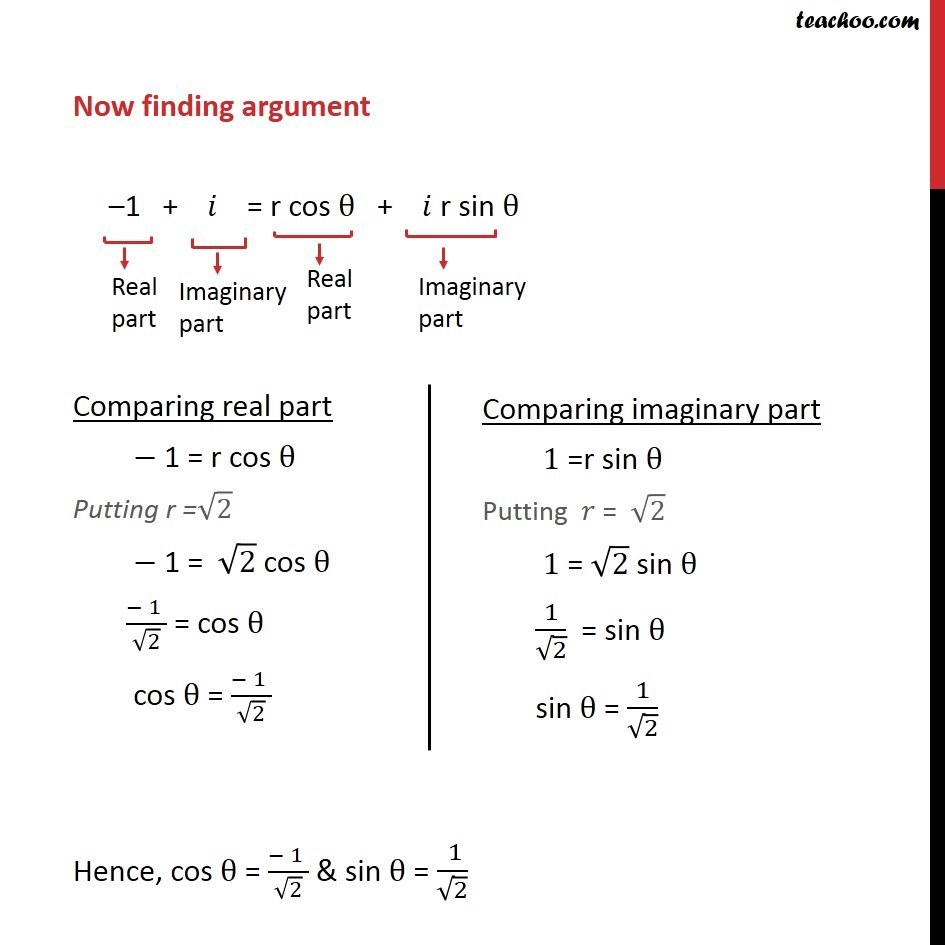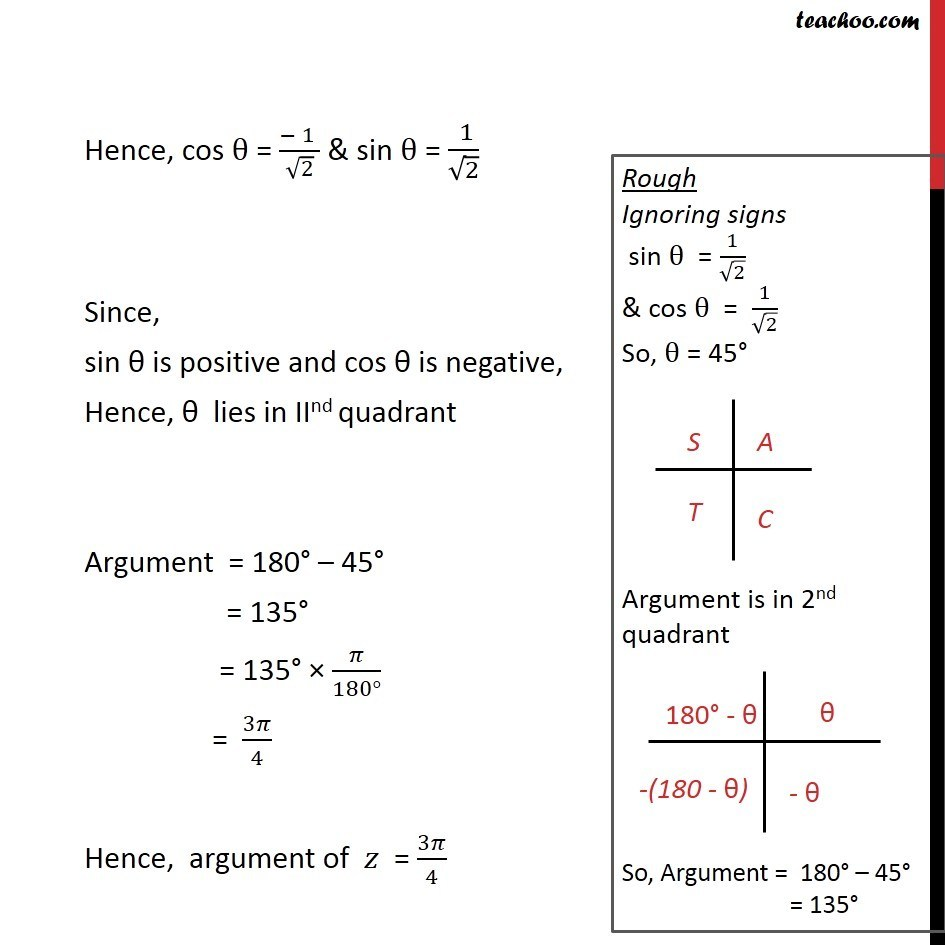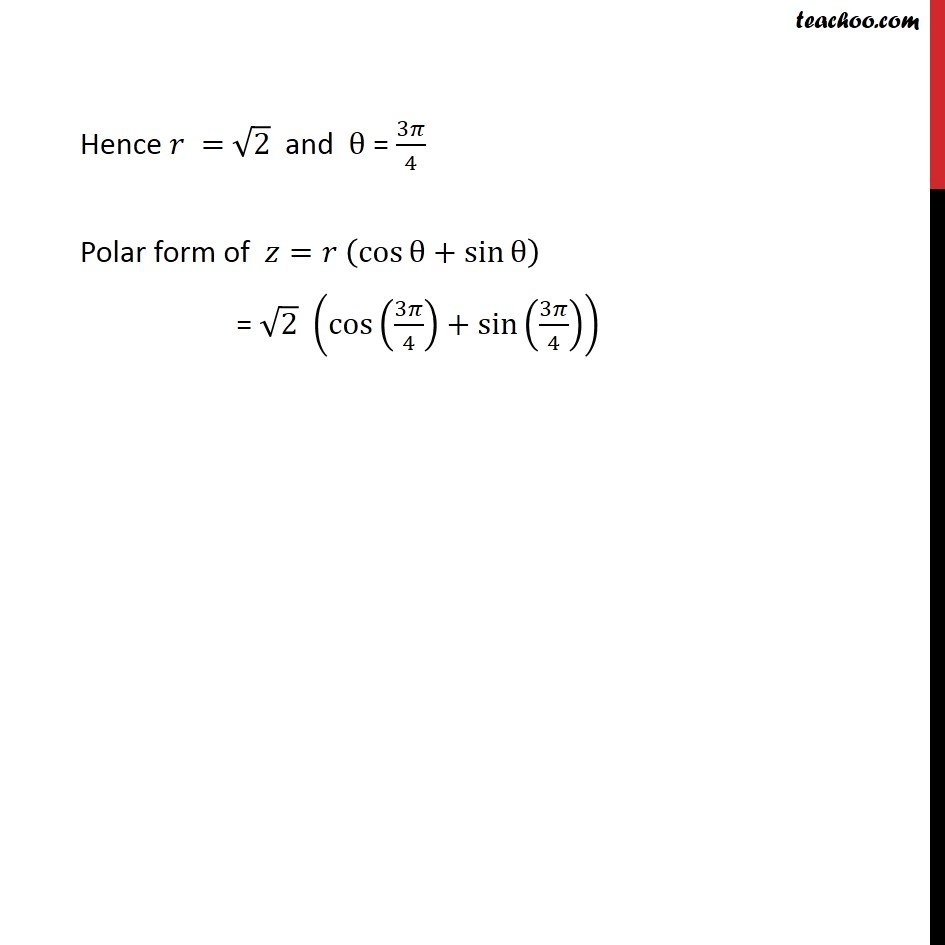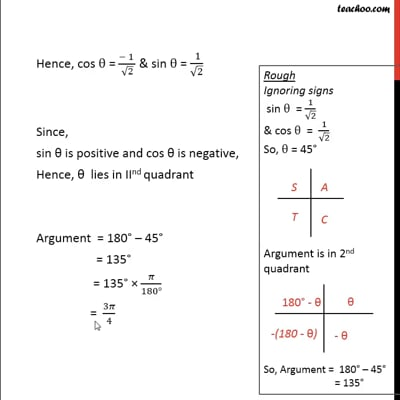This video is only available for Teachoo black users

Introducing your new favourite teacher - Teachoo Black, at only ₹83 per month

### Transcript

Misc 5 Convert the following in the polar form: (i) (1 + 7𝑖)/(2 − 𝑖)2 Let z = ( 1+7𝑖)/(2 − 𝑖)2 Using ( a – b)2 = a2 + b2 – 2ab = ((1 + 7𝑖))/((2)2+ (i)2 − 2 × 2 × 𝑖) = ( 1 + 7𝑖)/(4 + 𝑖2− 4𝑖) Putting 𝑖2 = -1 = (1 + 7𝑖)/(4 + ( −1 ) − 4𝑖) = (1 + 7𝑖 )/(4 − 1 − 4𝑖 ) = (1 + 7𝑖)/(3 − 4𝑖) Rationalizing the Same = (1 + 7𝑖)/(3 − 4𝑖) × (3 + 4𝑖)/(3 + 4𝑖) = ((1+7𝑖) (3 + 4𝑖 ))/((3 −4𝑖) (3 −4𝑖)) = (1 (3+4𝑖) +7𝑖 (3 + 4𝑖 ))/((3 −4𝑖) (3 + 4𝑖)) = (3 + 4𝑖 + 21𝑖 + 28𝑖2)/((3 − 4𝑖) (3 + 4𝑖)) = (3 + 25𝑖 + 28𝑖2)/((3 − 4𝑖) (3 + 4𝑖)) Using (a – b) (a + b) = a2 – b2 = (3 + 25𝑖 + 28𝑖2)/((3)2− (4𝑖)2) = (3 + 25𝑖 + 28𝑖2)/(9 − 16𝑖2) Putting 𝑖2 = 1 = (3 + 25𝑖 + 28 (−1 ))/(9 −16 ( −1 )) = (3 + 25𝑖 − 28)/(9 + 16) = (3 − 28 + 25𝑖)/25 = (− 25 + 25𝑖)/25 = ( 25 ( −1 + 𝑖 ))/25 = - 1 + 𝑖 Hence, z = – 1 + 𝑖 Let polar form be z = 𝑟 (cos⁡θ+𝑖sin⁡θ ) From (1) and (2) –1 + 𝑖 = r (cos θ + 𝑖 sin θ) –1 + 𝑖 = r cos θ + 𝑖 r sin θ Comparing real part − 1 = r cos θ Squaring both sides (− 1 )2 =( 𝑟 cos⁡θ )^2 1 = 𝑟2 cos2θ Adding (3) and (4) 1 + 1 = 𝑟2 cos2 θ + 𝑟2 sin2 θ 1 + 1 = r2 cos2 θ + r2 sin2 θ 2 = r2 ( cos2 θ + sin2 θ ) 2 = r2 × 1 2 = r2 √2 = r r = √2 Now finding argument –1 + 𝑖 = r cos θ + 𝑖 r sin θ Comparing real part − 1 = r cos θ Putting r =√2 − 1 = √2 cos θ (− 1 )/√2 = cos θ cos θ = (− 1 )/√2 Hence, cos θ = (− 1 )/√2 & sin θ = ( 1)/√2 Hence, cos θ = (− 1 )/√2 & sin θ = ( 1)/√2 Since, sin θ is positive and cos θ is negative, Hence, θ lies in IInd quadrant Argument = 180° – 45° = 135° = 135° × 𝜋/(180°) = 3𝜋/4 Hence, argument of 𝑧 = 3𝜋/4 Hence 𝑟 = √2 and θ = 3𝜋/4 Polar form of 𝑧=𝑟 (cos⁡θ+sin⁡θ ) = √2 (cos(3𝜋/4)+sin(3𝜋/4))